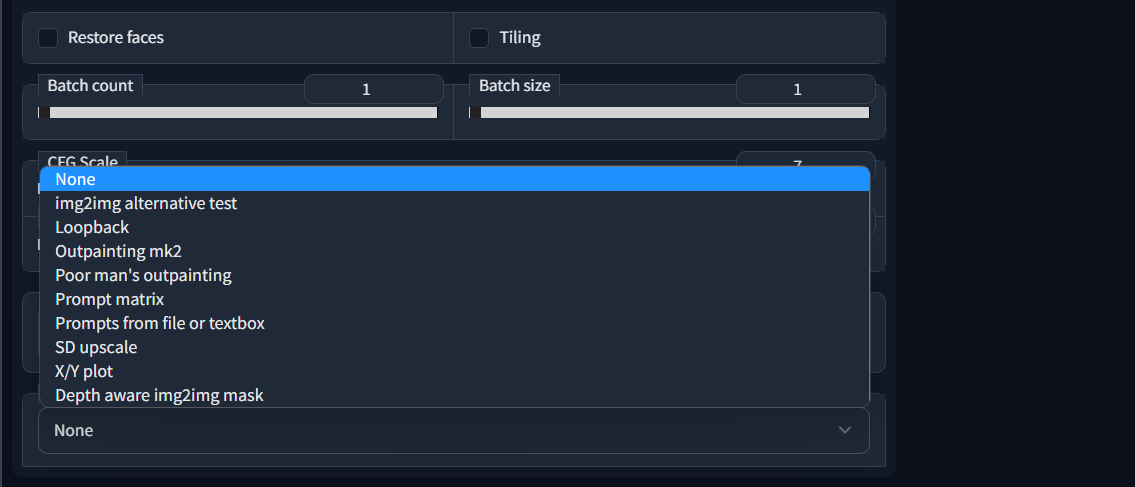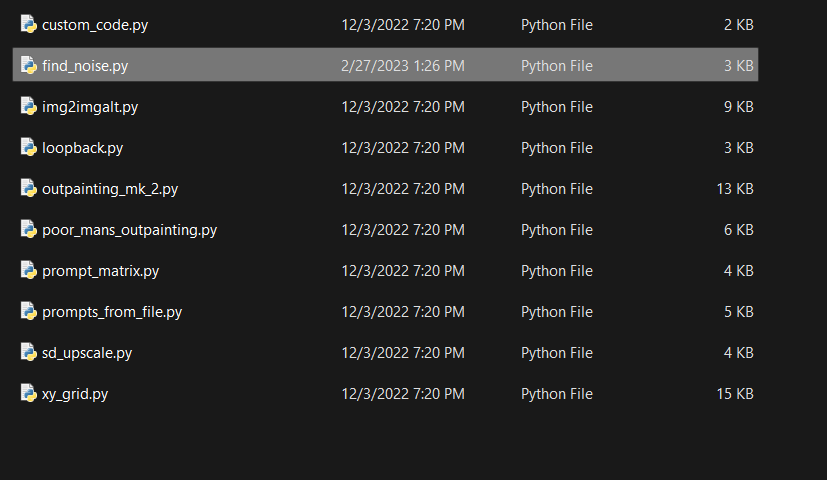Instantly share code, notes, and snippets.

# trygvebw/find_noise.py

Last active May 11, 2023 23:47
A "reverse" version of the k_euler sampler for Stable Diffusion, which finds the noise that will reconstruct the supplied image
This file contains bidirectional Unicode text that may be interpreted or compiled differently than what appears below. To review, open the file in an editor that reveals hidden Unicode characters. Learn more about bidirectional Unicode characters
 import torch import numpy as np import k_diffusion as K from PIL import Image from torch import autocast from einops import rearrange, repeat def pil_img_to_torch(pil_img, half=False): image = np.array(pil_img).astype(np.float32) / 255.0 image = rearrange(torch.from_numpy(image), 'h w c -> c h w') if half: image = image.half() return (2.0 * image - 1.0).unsqueeze(0) def pil_img_to_latent(model, img, batch_size=1, device='cuda', half=True): init_image = pil_img_to_torch(img, half=half).to(device) init_image = repeat(init_image, '1 ... -> b ...', b=batch_size) if half: return model.get_first_stage_encoding(model.encode_first_stage(init_image.half())) return model.get_first_stage_encoding(model.encode_first_stage(init_image)) def find_noise_for_image(model, img, prompt, steps=200, cond_scale=0.0, verbose=False, normalize=True): x = pil_img_to_latent(img, batch_size=1, device='cuda', half=True) with torch.no_grad(): with autocast('cuda'): uncond = model.get_learned_conditioning(['']) cond = model.get_learned_conditioning([prompt]) s_in = x.new_ones([x.shape]) dnw = K.external.CompVisDenoiser(model) sigmas = dnw.get_sigmas(steps).flip(0) if verbose: print(sigmas) with torch.no_grad(): with autocast('cuda'): for i in trange(1, len(sigmas)): x_in = torch.cat([x] * 2) sigma_in = torch.cat([sigmas[i - 1] * s_in] * 2) cond_in = torch.cat([uncond, cond]) c_out, c_in = [K.utils.append_dims(k, x_in.ndim) for k in dnw.get_scalings(sigma_in)] if i == 1: t = dnw.sigma_to_t(torch.cat([sigmas[i] * s_in] * 2)) else: t = dnw.sigma_to_t(sigma_in) eps = model.apply_model(x_in * c_in, t, cond=cond_in) denoised_uncond, denoised_cond = (x_in + eps * c_out).chunk(2) denoised = denoised_uncond + (denoised_cond - denoised_uncond) * cond_scale if i == 1: d = (x - denoised) / (2 * sigmas[i]) else: d = (x - denoised) / sigmas[i - 1] dt = sigmas[i] - sigmas[i - 1] x = x + d * dt if normalize: return (x / x.std()) * sigmas[-1] else: return x

### newsbubbles commented Sep 14, 2022

What is the k_diffusion library you are using for this? Can I get a github or pypi?

### parsec501 commented Sep 14, 2022

What is the k_diffusion library you are using for this? Can I get a github or pypi?

It's an alternative sampler

https://github.com/crowsonkb/k-diffusion

### newsbubbles commented Sep 14, 2022

What is the k_diffusion library you are using for this? Can I get a github or pypi?

It's an alternative sampler

https://github.com/crowsonkb/k-diffusion

Thanks a lot.

### thedarkzeno commented Oct 7, 2022

is there a diffusers compatible version?

### richservo commented Oct 22, 2022 • edited

Got it working. Thank you for this! Getting amazing results now!

### Ninja-Ferret commented Feb 27, 2023

how do you use this code / where do you put it in the stable diffusion folder?

### parsec501 commented Feb 27, 2023

how do you use this code / where do you put it in the stable diffusion folder?

I think you need to copy the code into a .py file and copy that .py file into /scripts in the automatic1111 root. Then it should show up in the "Scripts" dropdown in the web ui

### Ninja-Ferret commented Feb 27, 2023 • edited

how do you use this code / where do you put it in the stable diffusion folder?

I think you need to copy the code into a .py file and copy that .py file into /scripts in the automatic1111 root. Then it should show up in the "Scripts" dropdown in the web ui

thank you for replying! I did this method but I don't see it in the "Scripts" dropdown in the web ui. It's supposed to be in "C:\Users\user\AI\stable-diffusion-webui\scripts" right?### sungmo96 commented Mar 21, 2023

Hi, I am getting a run time error saying : "RuntimeError: Input type (c10::Half) and bias type (float) should be the same". Anyone else getting the same error? Not so sure how I can fix this.